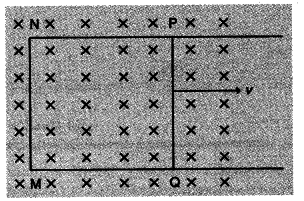# A rectangular loop PQMN

A rectangular loop PQMN with movable arm PQ of length 10 cm and resistance Q is placed in a uniform magnetic field of 0.1 Tesla perpendicular to the plane of the loop as shown in the figure.The resistances of the arms MN, NP and MQ are negligible. Calculate the (i) emf induced in the arm PQ and (ii) current induced in the loop when arm PQ is moved with velocity 20 m/s.(i) emf induced
e=Blv
$=0.1\times 10\times { 10 }^{ -2 }\times 20V\qquad$
=0.2 volt
(ii) Current in the loop
$i=\frac { e }{ R }$
$=\frac { 0.2 }{ 2 } A=0.1A$